# Impact of thermal radiation and Joule heating on MHD mixed convection flow of a Jeffrey fluid over a stretching sheet using homotopy analysis method

Impact of thermal radiation and Joule heating on MHD mixed convection flow of a Jeffrey fluid over a stretching sheet using homotopy analysis method

Prathi V. Kumar Shaik M. Ibrahim Giulio Lorenzini

Department of Mathematics, GITAM University, Visakhapatnam, Andhra Pradesh 530045, India

Department of Engineering and Architecture, University of Parma, Parco Area delle Scienze 181/A 43124 Parma, Italy

Corresponding Author Email:
giulio.lorenzini@unipr.it
Page:
978-986
|
DOI:
https://doi.org/ 10.18280/ijht.350434
|
Accepted:
|
Published:
31 December 2017
| Citation

OPEN ACCESS

Abstract:

A mathematical model has been developed to study the Joule heating effect on mixed convection MHD dissipative and radiative flow of an incompressible Jeffrey fluid due to a stretching sheet with power law heat flux, heat source/sink and suction. Similarity transformations are adopted to acquire ordinary differential equations from the governed partial differential equations. Series solutions are procured for these ordinary differential equations by admitting homotopy analysis method (HAM). A decisive way of convergence of series solutions is also provided. Characteristics of dissonant parameters on velocity, temperature, skin friction coefficient and Nusselt number are collected and discussed through graphs and tables. It is seen that the velocity enhances with an increase in Deborah number. Further the temperature is a depreciating function of Deborah number.

Keywords:

jeffrey fluid, thermal radiation, heat source, viscous dissipation, ham

1. Introduction

Fluids that do not obey the Newton’s law of motion are called non-Newtonian fluids. Analysis of boundary layer flow of non-Newtonian fluids due to a stretching sheet is absolutely a prominent field of research for many researchers. Such significance stems because of numerous important applications in various fields like biological sciences, geophysics, astrophysical bio-fluid and petroleum industries, chemical industries, etc. Many constituent relations of non-Newtonian fluids have been considered in the literature due to its versatile nature. Here we considered Jeffrey fluid model for non-Newtonian fluids. Jeffrey model is a rate type of non-Newtonian fluid which is capable of describing the characteristic of relaxation and retardation times. Kothandapani and Srinivas  studied Peristaltic transport of a Jeffrey fluid in an asymmetric channel in the presence of magnetic field. Tripathi et al.  analysed the peristaltic flow of the MHD Jeffrey fluid through a tube of finite length. Nadeem and Akbar  and Khan et al.  studied the effects of variable viscosity on an incompressible Jeffrey in an asymmetric channel. Krishna Murthy  has discussed two-dimensional MHD steady free convective mass transfer Couette flow of an electrically conducting Jeffrey fluid in a channel through a porous medium in the presence of heat source and chemical reaction. Akbar et al.  have examined the instinct system of cilia motion with magnetic field and slip for Jeffrey fluid model in a symmetric channel by long-wavelength and low Reynolds approximation. Sandeep et al.  have investigated stagnation point flow of MHD Jeffrey nanofluid over a stretching surface with heat and mass transfer in the presence of non-uniform heat source or sink and chemical reaction using similarity transformation. Qasim  studied heat source/sink effects on heat and mass transfer in a Jeffrey fluid over a stretching sheet. Jena et al.  reported the combined effect of heat and mass transfer in Jeffrey fluid flow through porous medium over a stretching sheet subject to transverse magnetic field in the presence of heat source/sink.

Viscous dissipation and Joule heating concepts play a great role in distinct fields like geophysical flow, nuclear engineering, etc. Alim et al.  analysed the Joule heating effect on MHD free convection flow from a vertical flat plate. Eldahab and Aziz  examined the viscous dissipation and Joule heating effects on MHD-free convection flow past a semi-infinite vertical flat plate by taking Hall and ion-slip currents. Amin and Mohammadein  analysed the Hiemenz Flow of a micropolar fluid. Newtonian heating and Joule heating effects on the magnetohydrodynamic (MHD) flow of Jeffrey liquid persuaded by a nonlinear radially stretched sheet were studied by Hayat et al. . Unsteady MHD convective flow in a vertical porous channel with viscous dissipation was discussed by Selvi and Muthuraj . Rudraswamy et al.  discussed the combined effect of viscous dissipation and Joule heating on three-dimensional flow and heat transfer of a Jeffrey nanofluid. Hayat et al.  studied the boundary layer flow and heat transfer in third grade fluid over an unsteady permeable stretching sheet. Ahmed et al.  obtained the exact solution regarding convective heat transfer of a magnetohydrodynamic (MHD) Jeffrey fluid over a stretching sheet by taking joule and viscous dissipation, internal heat source/sink and thermal radiation effects in the flow region. Joule heating effect on MHD mixed convection flow of nanofluids between two concentric cylinders with chemical reaction was studied by Srinivasacharya and Shafeeurrahman . Mabood et al.  proposed the radiation effects on Williamson nanofluid flow over a heated surface in presence of magnetohydrodynamics.

One of the most important methods for highly nonlinear problems is the homotopy analysis method (HAM) which was firstly employed by Liao [20, 21] for the nonlinear problems. This powerful method is being employed vastly by many researchers in different practical aspects of engineering and nonlinear problems. Heat transfer analysis in Darsian porous medium with radiation was carried out by Mabood and Khan . El-Aziz and Nabil  discussed the effect of time-dependent heat source/sink on heat transfer characteristics of the unsteady mixed convection flow over an exponentially stretching surface are investigated analytically. Liu et al.  analyzed the asymptotic behaviors of the steady state responses of a fractional van der Pol oscillator. Shehzad et al.  addressed the magnetohydrodynamic (MHD) radiative flow of an incompressible Jeffrey fluid over a linearly stretched surface. Hayat et al. [26, 27] analyzed the flow of Jeffrey fluid under various conditions. Ibrahim et al.  studied the mixed convection on MHD flow of Casson fluid over a nonlinearly permeable stretching sheet with thermal radiation, viscous dissipation, heat source/sink, chemical reaction and suction.

In this article a mathematical model has been proposed and analysed to study the influence of Joule heating on MHD Jeffery fluid flow over a moving porous stretching surface with thermal radiation. Homotopic algorithm is developed to find the expressions of velocity and temperature. Convergence of the developed series solutions is verified.

2. Mathematical Formulation

We consider a steady two-dimensional dissipative and radiative MHD flow of an incompressible, electrically conducting Jeffrey fluid over a moving porous stretching surface in the presence of joule heating and variable heat flux. A Cartesian coordinate system is chosen in such a way that x-axis is along the stretching surface and the y-axis perpendicular to it. The fluid fills the porous half space y>0. A constant magnetic field B0 is taken normal to the sheet. Two equal and opposite forces are applied along the x-axis so that the surface is stretched keeping the origin fixed and sheet issues from a thin slit. The magnetic Reynolds number is assumed small and so the induced magnetic field can be considered to be negligible. It is assumed that the speed of a point on the plate is proportional to its distance from the slit and the boundary layer approximations still applicable. It is also assumed that the prescribed heat flux at the stretching wall varies as the square of the distance from the origin. The flow configuration and coordinate system are as shown in Figure 1.

The constitutive equations for Jeffrey fluid can be written as $\tau=-p I+S$ , with S as the extra stress tensor and it defined by

$S=\frac{\mu}{1+\lambda}\left[R_{1}+\lambda_{1}\left(\frac{\partial R_{1}}{\partial t}+V \bullet \nabla\right) R_{1}\right]$

where $\tau$ is the Cauchy stress tensor, $\mu$  is the dynamic viscosity, $\lambda$  and $\lambda_{1}$  are the material parameters of Jeffrey fluid and $R_{1}$  is the Rivlin-Ericksen tensor defined by

$R_{1}=(\nabla V)+(\nabla V)^{\prime}$

Under the boundary layer approximations, the governing equations for conservation of mass, momentum, thermal energy and nanoparticle concentration of this problem can be expressed as

$\frac{\partial u}{\partial x}+\frac{\partial v}{\partial y}=0$          (1)

$u \frac{\partial u}{\partial x}+v \frac{\partial u}{\partial y}=\frac{v}{1+\lambda}\left\{\frac{\partial^{2} u}{\partial y^{2}}+\lambda_{1}\left\{\begin{array}{c}{u \frac{\partial^{3} u}{\partial x \partial y^{2}}+v \frac{\partial^{3} u}{\partial y^{3}}} \\ {-\frac{\partial u}{\partial x} \frac{\partial^{2} u}{\partial y^{2}}+\frac{\partial u}{\partial y} \frac{\partial^{2} u}{\partial x \partial y}}\end{array}\right\}\right\}$ ,

$+g \beta_{T}\left(T-T_{\infty}\right)-\frac{\nu}{K^{*}} u-\frac{\sigma B_{0}^{2}}{\rho} u$     (2)

$u \frac{\partial T}{\partial x}+v \frac{\partial T}{\partial y}=\frac{k}{\rho c_{p}} \frac{\partial^{2} T}{\partial y^{2}}+\frac{v}{c_{p}}\left(\frac{\partial u}{\partial y}\right)^{2}-\frac{1}{\rho c_{p}} \frac{\partial q_{r}}{\partial y}$

$+\frac{1}{\rho c_{p}} Q_{0}\left(T_{\infty}-T\right)+\frac{\sigma B_{0}^{2}}{\rho c_{p}} u^{2}$          (3)

Subject to the boundary conditions

$\begin{array}{ll}{u=c x, v=-v_{0}, \frac{\partial T}{\partial y}=B x^{2}} & {\text { at } y=0} \\ {u \rightarrow 0, u^{\prime} \rightarrow 0, \quad T \rightarrow T_{\infty}} & {\text { as } y \rightarrow \infty}\end{array}$         (4)

where u and v are the velocity components in x and y directions, $\mathrm{v}=\frac{\mu}{\rho}$ is the kinematic viscosity, $\rho$  is the fluid density, $\lambda$  is the ratio of relaxation and retardation times, $\lambda_{1}$  is the relaxation time, $\sigma$  is the electrical conductivity of the fluid, $K^{*}$  is the permeability of porous medium, $B_{0}$  is the transverse magnetic field strength, $\mu$  is the coefficient of viscosity, $c_{p}$  is the specific heat at constant pressure, T is the temperature in the boundary layer, k is the thermal conductivity, $Q_{0}$ is the heat source coefficient, $q_{r}$ is the radiative heat flux, $T_{\infty}$  is the free stream temperature, c is the proportionality constant, B is the stretching rate and it is a positive constant. The thermal boundary conditions depend on the type of heating process under consideration. Here we consider heating processes as variable surface heat flux varying with the distance.

## 1.png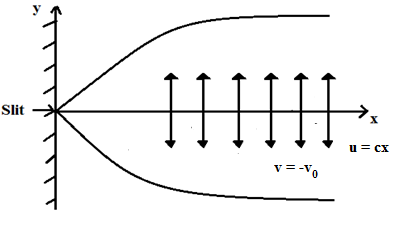Figure 1. Physical configuration

Following Rosseland approximation, the radiative heat flux is

$q_{r}=-\frac{4 \sigma^{*}}{3 k^{*}} \frac{\partial T^{4}}{\partial y}$

where $\sigma^{*}$  is the Stefan-Boltzman constant and $k^{*}$  is the mean absorption coefficient. Further, we assume that the temperature difference within the flow is such that $T^{4}$ is expressed as a linear function of temperature. Hence, expanding $T^{4}$  in Taylor series about $T_{\infty}$  and neglecting higher order terms, we obtain $T^{4} \cong 4 T_{\infty}^{3} T-3 T_{\infty}^{4}$ .

Now, we introduce the following similarity transformations:

$u=c x f^{\prime}(\xi), \xi=y \sqrt{\frac{c}{v}}, v=-\sqrt{c \nu} f(\xi)$

$T=T_{w}=T_{\infty}+B x^{2} \sqrt{\frac{\nu}{c}} \theta(\xi), \theta(\xi)=\frac{T-T_{\infty}}{T_{w}-T_{\infty}}$.    (5)

Equation (1) is automatically satisfied and the equations Eq. (2) to Eq. (4) cab be written as

$f^{\prime \prime \prime}+\beta\left(f^{n^{2}}-f f^{\prime \prime \prime \prime}\right)+(1+\lambda)\left(f f^{\prime \prime}-f^{\prime 2}\right)$

$-(1+\lambda)\left(M+\frac{1}{K}\right) f^{\prime}+(1+\lambda) G r \theta=0$         (6)

$\left(1+\frac{4}{3} R\right) \theta^{\prime \prime}+\operatorname{Pr} f \theta^{\prime}-2 \operatorname{Pr} f^{\prime} \theta$

$+\operatorname{Pr} E c\left(f^{\prime \prime 2}+M f^{\prime 2}\right)-\operatorname{Pr} Q \theta=0$         (7)

The boundary conditions are

$\begin{array}{cc}{f(0)=S, f^{\prime}(0)=1,} & {\theta^{\prime}(0)=1} \\ {f^{\prime}(\infty)=0, f^{\prime \prime}(\infty)=0} & {\theta(\infty)=0}\end{array}$        (8)

where prime denotes differentiation with respect to $\xi$ , $\beta=\lambda_{1} c$  is the Deborah number, $K=\frac{v}{c K^{*}}$  is the permeability parameter, $M=\frac{\sigma B_{0}^{2}}{\rho c}$  is the magnetic parameter, $G r=\frac{g \beta_{T}\left(T_{w}-T_{\infty}\right)}{c^{2} x}$  is the Grashoff number, $S=\frac{v_{0}}{\sqrt{v c}}$  is the suction parameter, $P r=\frac{\rho C_{p} v}{k}$  is the Prandtl number, $R=\frac{4 \sigma^{*} T_{\infty}^{3}}{k^{*} k}$  is the radiation parameter, $Q=\frac{Q_{0}}{\rho C_{p} c}$  is the heat generation parameter, $E c=\frac{c^{2} x^{2}}{c_{p}\left(T_{w}-T_{\infty}\right)}$  is the Eckert number.

Expressions for skin friction coefficient $C_{f}$  and local Nusselt number $N u_{x}$  are

$C_{f}=\frac{\tau_{w}}{\rho u_{w}^{2}}$  and $N u_{x}=\frac{x q_{w}}{k\left(T_{w}-T_{\infty}\right)}$ where $\tau_{w}=\mu\left(\frac{\partial u}{\partial y}\right)_{y=0}$   $q_{w}=\left(-\left(k+\frac{16 \sigma^{*} T_{\infty}^{3}}{3 k^{*}}\right)\left(\frac{\partial T}{\partial y}\right)\right)_{y=0}$ .

Using $\tau_{w}$  and $q_{w}$ , we have

$R e_{x}^{1 / 2} C_{f}=\frac{1+\beta}{1+\lambda} f^{\prime \prime}(0)$  and $R e_{x}^{-1 / 2} N u_{x}=-\frac{\left(1+\frac{4}{3} R\right)}{\theta(0)}$  where $R e_{x}=\frac{c x^{2}}{v}$  is the local Reynolds number.

3. Ham

For the homotopic solutions of the equations (6) and (7) subjected to the boundary conditions (8), we choose

$f_{0}(\xi)=S+1-e^{-\xi}$

$\theta_{0}(\xi)=-e^{-\xi}$

$L_{1}(f)=f^{\prime \prime}-f^{\prime}$

$L_{2}(\theta)=\theta^{\prime \prime}-\theta$

with the following properties

$L_{1}\left(C_{1}+C_{2} e^{\xi}+C_{3} e^{-\xi}\right)=0$

$L_{2}\left(C_{4} e^{\xi}+C_{5} e^{-\xi}\right)=0$

where $C_{i}(i=1 \text { to } 5)$  are the arbitrary constants.

We construct the zeroth-order deformation equations as

$(1-p) L_{1}\left(f(\xi ; p)-f_{0}(\xi)\right)=p \hbar_{1} N_{1}[f(\xi ; p), \theta(\xi ; p)]$      (9)

$(1-p) L_{2}\left(\theta(\xi ; p)-\theta_{0}(\xi)\right)=p \hbar_{2} N_{2}[f(\xi ; p), \theta(\xi ; p)]$      (10)

subject to the boundary conditions

$\begin{array}{l}{f(0 ; p)=S, f^{\prime}(0 ; p)=1, f^{\prime}(\infty ; p)=0, f^{\prime \prime}(\infty ; p)=0} \\ {\theta^{\prime}(0 ; p)=1,} & {\theta(\infty ; p)=0}\end{array} \}$      (11)

$N_{1}[f(\xi ; p)]=\frac{\partial^{3} f(\xi ; p)}{\partial \xi^{3}}+\beta\left(\begin{array}{c}{\left(\frac{\partial^{2} f(\xi ; p)}{\partial \xi^{2}}\right)^{2}} \\ {-f(\xi ; p) \frac{\partial^{4} f(\xi ; p)}{\partial \xi^{4}}}\end{array}\right)$

$+(1+\lambda)\left(f(\xi ; p) \frac{\partial^{2} f(\xi ; p)}{\partial \xi^{2}}-\left(\frac{\partial f(\xi ; p)}{\partial \xi}\right)^{2}\right)$

$-(1+\lambda)\left(M+\frac{1}{K}\right) \frac{\partial f(\xi ; p)}{\partial \xi}+(1+\lambda) \operatorname{Gr} \theta(\xi ; p)$      (12)

$N_{2}[f(\xi ; p), \theta(\xi ; p)]=\left(1+\frac{4}{3} R\right) \frac{\partial^{2} \theta(\xi ; p)}{\partial \xi^{2}}$

$+P r\left(f(\xi ; p) \frac{\partial \theta(\xi ; p)}{\partial \xi}-2 \frac{\partial f(\xi ; p)}{\partial \xi} \theta(\xi ; p)\right)$

$+P r E c\left(\left(\frac{\partial^{2} f(\xi ; p)}{\partial \xi^{2}}\right)^{2}+M\left(\frac{\partial f(\xi ; p)}{\partial \xi}\right)^{2}\right)-\operatorname{Pr} Q \theta(\xi ; p)$   (13)

where $p \in[0,1]$  is the embedding parameter, $\hbar_{1}$ and $\hbar_{2}$ are the non-zero auxiliary parameters.

When $p=0$  and $p=1$, we obtain

$\begin{array}{ll}{f(\xi ; 0)=f_{0}(\xi)} & {f(\xi ; 1)=f(\xi)} \\ {\theta(\xi ; 0)=\theta_{0}(\xi)} & {\theta(\xi ; 1)=\theta(\xi)}\end{array}$       (14)

Thus, as $p$  increases from 0 to 1 then $f(\xi ; p)$  and $\theta(\xi ; p)$ vary from initial approximations to the exact solutions of the original nonlinear differential equations.

Now, with the help of Taylor’s series, we can write

$f(\xi ; p)=f_{0}(\xi)+\sum_{m=1}^{\infty} f_{m}(\xi) p^{m}$        (15)

$\theta(\xi ; p)=\theta_{0}(\xi)+\sum_{m=1}^{\infty} \theta_{m}(\xi ;) p^{m}$       (16)

where

$f_{m}(\xi)=\frac{1}{m !}\left.\frac{\partial^{m} f(\xi ; p)}{\partial p^{m}}\right|_{p=0}$

$\theta_{m}(\xi)=\frac{1}{m !}\left.\frac{\partial^{m} \theta(\xi ; p)}{\partial p^{m}}\right|_{p=0}$             (17)

If the initial approximations, auxiliary linear operators and non-zero auxiliary parameters are chosen in such a way that the series Eq. (15) and Eq. (16) are convergent at $p=1$  then

$f(\xi)=f_{0}(\xi)+\sum_{m=1}^{\infty} f_{m}(\xi)$         (18)

$\theta(\xi)=\theta_{0}(\xi)+\sum_{m=1}^{\infty} \theta_{m}(\xi)$          (19)

The mth-order deformation equations are follows

$L_{1}\left(f_{m}(\xi)-\chi_{m} f_{m-1}(\xi)\right)=\hbar_{1} R_{m}^{f}(\xi)$              (20)

$L_{2}\left(\theta_{m}(\xi)-\chi_{m} \theta_{m-1}(\xi)\right)=\hbar_{2} R_{m}^{\theta}(\xi)$          (21)

with the following boundary conditions

$\begin{array}{ll}{f_{m}(0)=0,} & {f_{m}^{\prime}(0)=0, \quad f_{m}^{\prime}(\infty)=0, f_{m}^{\prime \prime}(\infty)=0} \\ {\theta_{m}^{\prime}(0)=0,} & {\theta_{m}(\infty)=0}\end{array}$       (22)

where

$R_{m}^{f}(\xi)=f_{m-1}^{\prime \prime \prime}+\beta\left(\sum_{i=0}^{m-1} f_{m-1-i}^{\prime \prime} f_{i}^{\prime \prime}-\sum_{i=0}^{m-1} f_{m-1-i} f_{i}^{\prime \prime \prime \prime}\right)$

$+(1+\lambda)\left(\sum_{i=0}^{m-1} f_{m-1-i} f_{i}^{\prime \prime}-\sum_{i=0}^{m-1} f_{m-1-i}^{\prime} f_{i}^{\prime}\right)$

$-(1+\lambda)\left(M+\frac{1}{K}\right) f_{m-1}^{\prime}+(1+\lambda) G r \theta_{m-1}$        (23)

$R_{m}^{\theta}(\xi)=\left(1+\frac{4}{3}\right) \theta_{m-1}^{\prime \prime}+\operatorname{Pr}\left(\begin{array}{l}{\sum_{i=0}^{m-1} f_{m-1-i} \theta_{i}^{\prime}} \\ {-2 \sum_{i=0}^{m-1} f_{m-1-i}^{\prime} \theta_{i}}\end{array}\right)$$+\operatorname{Pr} E c\left(\sum_{i=0}^{m-1} f_{m-1-i}^{\prime \prime} f_{i}^{\prime \prime}+M \sum_{j=0}^{m-1} f_{m-1-i}^{\prime} f_{i}^{\prime}\right)-\operatorname{Pr} Q \theta_{m-1}$         (24)

$\chi_{m}=\left\{\begin{array}{ll}{0,} & {m \leq 1} \\ {1,} & {m>1}\end{array}\right.$         (25)

The general solution can be written as

$f_{m}(\xi)=f_{m}^{*}(\xi)+C_{1}+C_{2} e^{\xi}+C_{3} e^{-\xi}$

$\theta_{m}(\xi)=\theta_{m}^{*}(\xi)+C_{4} e^{\xi}+C_{5} e^{-\xi}$          (26)

where $f_{m}^{*}(\xi)$ and $\theta_{m}^{*}(\eta)$ are the special solutions of mth order deformation equations.

4. Convergence of Ham

The convergence of the acquired solutions depends upon the non-zero auxiliary parameters $\hbar_{1}$ and $\hbar_{2}$. To acquire the relevant values for these parameters, $\hbar$ -curves are portrayed in Figure 2. From the figure, it is scrutinized that the plausible region of the parameters is about $[-1.0,0.0]$ . For $\hbar_{1}=\hbar_{2}=-0.45$ , the series solutions are convergent in the whole region of $\xi$ . Table 1 displays the convergence of the method when $\beta=0.1, M=0.5, \lambda=0.2, G r=0.1$ , K=10, S=0.1, R=0.1, Pr=1.0, Ec=0.2, Q=0.2.

## 2.png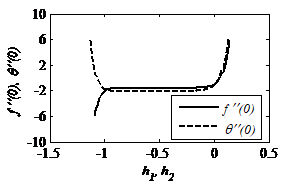Figure 2. $\hbar$ -curves of $f^{\prime \prime}(0)$ and $\theta^{\prime \prime}(0)$ for $15^{\text { th }}$ order approximation

Table 1. Convergence of HAM solution for different orders of approximations

 Order $-f^{\prime \prime}(0)$ $-\theta^{\prime \prime}(0)$ 5 1.572939 2.055736 10 1.579667 2.108476 15 1.579621 2.110438 20 1.579618 2.110489 25 1.579618 2.110490 30 1.579618 2.110490 35 1.579618 2.110490 40 1.579618 2.110490 45 1.579618 2.110490 50 1.579618 2.110490 55 1.579618 2.110490 60 1.579618 2.110490
5. Results and Discussion

The desire of this study is to construe the outcomes of various parameters such as Deborah number (β), ratio of relaxation and retardation times (λ), magnetic parameter (M), permeability parameter (K), Grashof number (Gr), suction parameter (S), radiation parameter (R), Prandtl number (Pr), Eckert number (Ec) and heat source parameter (Q) on velocity, temperature, skin friction coefficient and Nusselt number.

In this study following default parameter values are undertaken for computations:

$\beta=0.1, M=0.5, \lambda=0.2, G r=0.1, K=10, S=0.5, \mathrm{R}=0.1$

$\mathrm{Pr}=1.0, E c=0.2, \mathrm{Q}=0.2$

The impact of ratio of relaxation to retardation times $\lambda$  on the velocity and temperature profiles are illustrated in Figures 3 and 4. It is observed that the increase of $\lambda$ causes the devaluation of both boundary layer thickness and velocity of the fluid. These effects are ample solider close to the surface of the sheet. This confirms that the increase of $\lambda$  gives the field retardation and which effects to prevent the increase of the fluid motion. Further, maximum temperature and thinnest thermal boundary layer thickness is noticed. Thus, an increase in $\lambda$  implies to an increase in relaxation time and decrease in retardation time. This change in relaxation and retardation times elucidates the higher temperature and thicker thermal boundary layer thickness.

Figures 5 and 6 describe the effect of Deborah number β  on velocity and temperature. Velocity accelerates with β and opposite phenomena is noticed in the case of temperature profiles. This is due to the direct proportionality of β retardation time. Due to the Lorentz force admitted by the magnetic field in the flow region, the velocity deteriorates and temperature enhances with the magnetic parameter M . This is portrayed in Figures 7 and 8.

Figure 9 depicts the effect of permeability of the porous medium parameter K  on the velocity distribution. It is obvious that as K  increase, the velocity increases along the boundary layer. Also, it is expected that, an increase in the permeability of the porous medium leads to the rise in the fluid flow. Thus, the holes of the porous medium become larger and then the resistivity of the medium may be neglected.Figure 10 depicts that an increase in thermal buoyancy parameter leads to a depreciation in the velocity profile. Figure 11 illustrates that an increase in the thermal buoyancy parameter leads to an increase in the temperature profile and thermal boundary layer thickness.

Figures 12 and 13 display the velocity profile and temperature profile for the suction parameter S  on the velocity and temperature. The velocity, boundary layer thickness, temperature and thermal boundary layer thickness are decreasing function of S.

As R  increases, heat energy will be released to the fluid as a result amplification takes place in the temperature distribution with R . This is delivered in Figure 14. In case of higher Prandtl values the diffusion of heat away from the heated surface is very slow when compared to the smaller Prandtl values. Hence temperature depreciates with the acceleration of Prandtl number Pr as delineated in Figure 15.

The positive Eckert numbers implies cooling of the sheet hence temperature declines with Eckert number. This is shown in Figure 16. Physically $Q>0$  indicates $T_{w}>T_{\infty}$  which entails the supply of heat to the flow from the wall. Therefore temperature declines with heat source parameter as shown in Figure 17.

Tables 2 and 3 show superb correlation of present results with the previous results for $-f^{\prime \prime}(0)$ . Table 4 demonstrates the impact of effective parameters on skin friction coefficient and Nusselt number. From the table, it is clear that skin friction is increasing with Deborah number  $\beta$  and opposite result is observed in the case of Nusselt number. Skin friction coefficient decreases with $\lambda$ , $E c$  and increases with $R$ . Nusselt number rises with $\lambda$  and decreases with $E_{C}, R$ .

## 3.png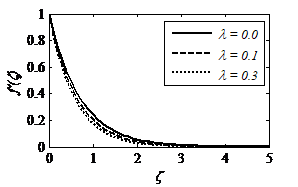Figure 3. Effect of $\lambda$ on $f^{\prime}(\xi)$

## 4.png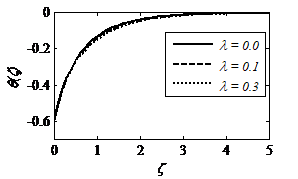Figure 4. Effect of $\lambda$ on $\theta(\xi)$

## 5.png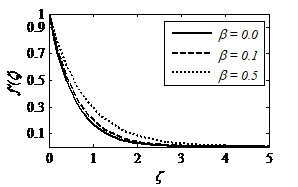Figure 5. Effect of $\beta$ on $f^{\prime}(\xi)$

## 6.png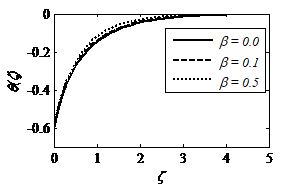Figure 6. Effect of $\beta$ on $\theta(\xi)$

## 7.pngFigure 7. Effect of $M$ on $f^{\prime}(\xi)$

## 8.png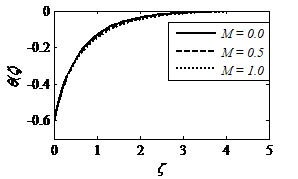Figure 8. Effect of $M$ on $\theta(\xi)$

## 9.pngFigure 9. Effect of $K$ on $f^{\prime}(\xi)$

## 10.png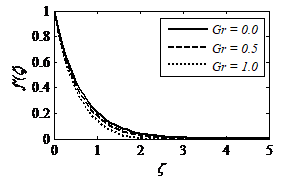Figure 10. Effect of Gr on $f^{\prime}(\xi)$

## 11.png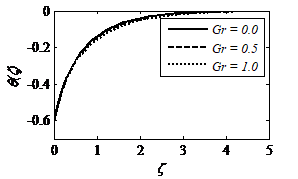Figure 11. Effect of Gr on $\theta(\xi)$

## 12.png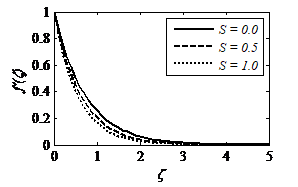Figure 12. Effect of $S$ on $f^{\prime}(\xi)$

## 12.pngFigure 13. Effect of $S$ on $\theta(\xi)$

## 14.png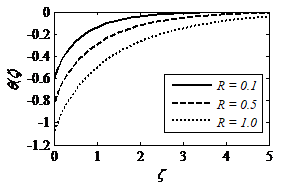Figure 14. Effect of $R$ on $\theta(\xi)$

## 15.png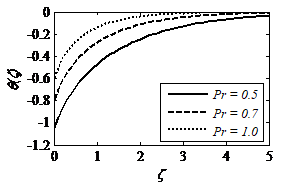Figure 15. Effect of Pr on $\theta(\xi)$

## 16.png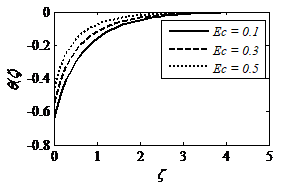Figure 16. Effect of $E c$ on $\theta(\xi)$

## 17.pngFigure 17. Effect of $Q$ on $\theta(\xi)$

Table 2. Comparison of $-f^{\prime \prime}(0)$  when $\beta=\lambda=S=G r=0.0, K \rightarrow \infty$

 M Chen  Harish Babu and Satya Narayana  HAM 0.0 1.00000 1.00000 1.000000 0.5 1.22425 1.22479 1.224745 1.0 1.41421 1.41432 1.414214 1.5 1.58114 1.58115 1.581139 2.0 1.73205 1.73225 1.732051

Table 3. Comparison of $-\theta(0)$  when $\beta=G r=\lambda=\mathrm{S}=R=E c=Q=0.0, \mathrm{Pr}=1.0, K \rightarrow \infty$

 M Turkyilmazoglu  Kayalvizhi et al.  HAM 0.0 0.750000 0.75000000 0.750000 1.0 0.822522 0.82252217 0.822519

Table 4. Values of skin friction coefficient and Nusselt number for different parameters when $M=0.5, K=10, G r=0.1, S=0.5, \mathrm{Pr}=1.0, Q=0.2$ .

 $\lambda$ $\beta$ $R$ $E c$ $C_{f} \operatorname{Re}_{x}^{1 / 2}$ $N u_{x} \mathrm{Re}_{x}^{-1 / 2}$ 0.0 0.1 0.1 0.2 -1.563541 1.941470 0.2 0.1 0.1 0.2 -1.447983 1.928555 0.4 0.1 0.1 0.2 -1.358119 1.918286 0.2 0.2 0.1 0.2 -1.458568 1.938243 0.2 0.3 0.1 0.2 -1.478294 1.946607 0.2 0.4 0.1 0.2 -1.503133 1.953930 0.2 0.1 0.0 0.2 -1.444229 1.892574 0.2 0.1 0.3 0.2 -1.455853 1.996502 0.2 0.1 0.5 0.2 -1.464164 2.057330 0.2 0.1 0.1 0.1 -1.450512 1.789266 0.2 0.1 0.1 0.3 -1.445469 2.090476 0.2 0.1 0.1 0.5 -1.440481 2.508594
6. Conclusions

The present study describes the boundary layer flow of a dissipative and radiative MHD flow of Jeffrey fluid with heat effects. Series solutions are procured for these ordinary differential equations by admitting homotopy analysis method (HAM). The present results are validated by comparing with available literature and notied favorable agreement.

The main observations of this study are as follows:

• The Deborah number  $\beta$  has opposite effects on skin friction and Nusselt numbers.
• The Nusselt number decreases in view of increase in $E c$ .
• The effect of Deborah number and parameter $\lambda$  on the velocity is quite opposite.

The heat generation parameter leads to a decrease in temperature profiles.

Nomenclature
 $u, v$ velocity components in $x, y$  directions $x, y$ axian and normal coordinates $k$ thermal conductivity of the fluid, W. m-1. k-1 B dimensional temperature coefficient B0 magnetic induction, T c stretching rate, s-1 Cf skin friction coefficient cp specific heat at constant pressure, J. kg-1. K-1 k* mean absorption coefficient Q0 heat source coefficient qr radiative heat flux, W. m-1 qw surface heat flux Rex local Reynoldsnumber T fluid temperature, K Tw wall temperature on sheet y=0, K $T_{\infty}$ temperature far away from wall, K $v_{0}$ suction velocity across stretching sheet M magnetic field, N. m-1. A-1 Pr Ec Gr K R Nux S Q Prandtl number Eckert number Grashof number permeability of porous medium radiation parameter Nusselt number suction parameter heat source parameter Greek symbols $\beta$ Deborah number $\xi$ similarity variable $\lambda$ ratio of relaxation and retardation times $\lambda_{1}$ retardation time, s $\mu$ dynamic viscosity, Pa. s-1 $\mathbb{N}$ $\rho$ kinematic viscosity, m2. s-1 fluid density, kg. m-1 c electric conductivity, W. m-2. K-4 $\theta$ non-dimensional temperature $\tau$ Cauchy stress tensor $\tau_{w}$ shear stress along stretching sheet Subscripts $w$ sheet surface $\infty$ Infinity Superscript ' differentiation with respect to
References

 Kothandapani M., Srinivas S. (2008). Peristaltic transport of a Jeffrey fluid under the effect of magnetic field in an asymmetric channel, Int. J. Non Lin. Mech., Vol. 43, No. 9, pp. 915-924. DOI: 10.1016/j.ijnonlinmec.2008.06.009

 Tripathi D., Ali N., Hayat T., Chaube M.K., Hendi A.A. (2011). Peristaltic flow of MHD Jeffrey fluid through a finite length cylindrical tube, Appl. Math. Mech. Engl. Edit., Vol. 32, No. 10, pp. 1148-1160. DOI: 10.3879/j.issn.1000-0887.2011.10.002

 Nadeem S., Akbar N.S. (2009). Peristaltic flow of a Jeffrey fluid with variable viscosity in an asymmetric channel, Z. Naturforschung, Vol. 64, No. 11, pp. 713-722. DOI: 10.1515/zna-2009-1107

 Afsar Khan A., Ellahi R., Vafai K. (2012). Peristalic transport of a Jeffrey fluid with variable viscosity through a porous medium in an asymmetric channel, Advs in Math. Phys., Vol. 2012, pp. 1-15. DOI: 10.1155/2012/169642

 Krishna Murthy M. (2016). MHD Couette flow of Jeffrey fluid in a porous channel with heat source and chemical reaction, Middle-East J. Sci. Res., Vol. 24, No. 3, pp. 585-592. DOI: 10.5829/idosi.mejsr.2016.24.03.23067

 Akbar N.S., Khan Z.H., Nadeem S. (2016). Influence of magnetic field and slip on Jeffrey fluid in a ciliated symmetric channel with metachronal wave pattern, J. Appl. Fluid Mech., Vol. 9, No. 2, pp. 565-572. DOI: 10.18869/acadpub.jafm.68.225.24665

 Sandeep N., Sulochana C., Animasaun I.L. (2015). Stagnation-point flow of a Jeffrey nano fluid over a stretching surface with induced magnetic field and chemical reaction, Int. J. Eng. Res. Afr., Vol. 20, pp. 93-111. DOI: 10.4028/www.scientific.net.jera.20.93

 Qasim M. (2013). Heat and mass transfer in a Jeffrey fluid over a stretching sheet with heat source/sink, Alexandria Eng. J., Vol. 52, No. 4, pp. 571-575. DOI: 10.1016/j.aej.2013.08.004

 Jena S., Misra S.R., Dash G.C. (2017). Chemical reaction effect on MHD Jeffrey fluid over a stretching sheet through porous media with heat generation/absorption, International Journal of Applied and Computational Mathematics, Vol. 3, No. 2, pp. 1225-1238. DOI: 10.1007/s40819-016-0173-8

 Alim M.A., Alam M.D., Mamun A. (2007). Joule heating effect on the coupling of conduction with magnetohydrodynamic free convection flow from a vertical plate, Nonlinear. Anal.Model. Control., Vol. 12, No. 3, pp. 307-316.

 Eldahab E.M.A., El. Azia M.A. (2005). Viscous dissipation and Joule heating effects on MHD-free convection from a vertical plate with power-law variation in surface temperature in the presence of Hall and ion-slip currents, Appl. Math. Model., Vol. 29, No. 6, pp. 579-595. DOI: 10.1016/j.apm.2004.10.005

 EL-Amin M.F., Mohammadein A.A. (2005). Effects of viscous dissipation and Joule heating on magnetohydrodynamic Hiemenz flow of a micropolar fluid”, Heat Transf. Eng., Vol. 26, No. 6, pp. 75-81. DOI: 10.1080/0147630590951168

 Hayat T., Bashir G., Waqas M., Alsaedi A. (2016). MHD flow of Jeffrey liquid due to a nonlinear radially stretched sheet in presence of Newtonian heating, Results Phys., Vol. 6, pp. 817-823. DOI: 10.1016/j.rinp.2016.10.001

 Selvi R.K., Muthuraj R. (2017). MHD oscillatory flow of a Jeffrey fluid in a vertical porous channel with viscous dissipation, Ain. Shams. Eng. J. DOI: 10.1016/j.asej.2017.05.009

 Rudraswamy N.G., Ganesh Kumar K., Gireesha B.J., Gorla S.R. (2017). Combined effects of Joule heating and viscous dissipation on MHD three dimensional flow of a Jeffrey nanofluid, Journal of Nanofluid, Vol. 6, No. 2, pp. 300-310. DOI: 10.1166/jon.2017.1329

 Hayat T., Shafiq A., Alsaedi A. (2014). Effect of Joule heating and thermal radiation in flow of third grade fluid over a radiative surface, PLOS ONE, Vol. 9, No. 1, pp. e83153. DOI: 10.1371/journal.pone.0083153

 Ahmed J., Shahzad A., Khan M., Ali R. (2015). A note on convective heat transfer of an MHD Jeffrey fluid over a stretching sheet, AIP Advances, Vol. 5, No. 11, p. 117117. DOI: 10.1063/1.4935571

 Srinivasacharya D., Shafeeurrahman Md. (2017). Joule heating effect on entropy generation in MHD mixed convection flow of chemically reacting nanofluid between two concentric cylinders, International Journal of Heat and Technology, Vol. 35, No. 3, pp. 487-497. DOI: 10.18280/ijht.350305

 Mabood F., Ibrahim S.M, Lorenzini G., Lorenzini E. (2017). Radiation effects on Williamson nanofluid flow over a heated surface with magnetohydrodynamics, International Journal of Heat and Technology, Vol. 35, No. 1, pp. 196-204. DOI: 10. 18280/ijht.350126

 Liao S.J. (2012). Homotopy Analysis Method in Nonlinear Differential Equations, Springer & Higher Education Press, Heidelberg.

 Liao S.J. (1999). A uniformly valid analytic solution of two-dimensional viscous flow over a semi-infinite flat plate, J. Fluid. Mech., Vol. 385, pp. 101-128. DOI: 10.1017/s0022112099004292

 Mabood F., Khan W.A. (2014). Homotopy analysis method for boundary layer flow and heat transfer over a permeable flat plate in a darcian porous medium with radiation effect, J. Taiwan Inst. Chem. Eng., Vol. 45, No. 4, pp. 1217-1224. DOI: 10.1016/i.jtice.2014.03.019

  ABD El-Aziz M., Nabil T. (2015). Effect of time-dependent heat source/sink on slip flow and heat transfer from a stretching surface with homotopy analysis method, Meccanica, Vol. 50, No. 6, pp. 1467-1480. DOI: 10.1007/s11012-015-0113-4

 Liu Q.X., Liu J.K., Chen Y.M. (2016). Asymptotic limit cycle of fractional van der Pol oscillator by homotopy analysis method and memory-free principle, Appl. Math. Modell., Vol. 40, No. 4, pp. 3211-3220. DOI: 10.1016/j.apm.2015.10.005

 Shehzad S.A., Alsaedi A., Hayat T. (2013). Influence of thermophoresis and Joule heating on the radiative flow of Jeffrey fluid with mixed convection, Braz. J. Chem. Eng., Vol. 30, No. 4, pp. 897-908. DOI: 10.1590/s0104-66322013000400021

 Hayat T., Qayyum S., Imtiaz M., Alsaedi A. (2016). Three-dimensional rotating flow of Jeffrey fluid for Cattaneo-Christov heat flux model, AIP Advances, Vol. 6, No. 2, p. 025012. DOI: 10.1063/1.4942091

 Hayat T., Asad S., Alsaedi A., Alsaedi F.E. (2014). Radiative flow of Jeffrey fluid through a convectively heated stretching cylinder, J. Mech., Vol. 31, No. 1, pp. 69-78. DOI: 10.1017/jmech.2014.49

 Ibrahim S.M., Lorenzini G., Kumar P.V., Raju C.S.K. (2017). Influence of chemical reaction and heat source on dissipative MHD mixed convection flow of a Casson nanofluid over a nonlinear permeable stretching sheet, Int. J. Heat Mass Tran., Vol. 111, pp. 346-355. DOI: 10.1016/j.ijheatmasstransfer.2017.03.097

 Chen C.H. (2008). Effects of magnetic field and suction/injection on convection heat transfer of non-Newtonian power-law fluids past a power-law stretched sheet with surface heat flux, Int. J. Therm. Sci., Vol. 47, No. 7, pp. 954-961. DOI: 10.1016/j.ijthermalsci.2007.06.003

 Harish Babu D., Satya Narayana P.V. (2016). Joule heating effects on MHD mixed convection of a Jeffrey fluid over a stretching sheet with power law heat flux: A numerical study, J. Magn. Magn. Mater., Vol. 412, pp. 185-193. DOI: 10.1016/j.jmmm.2016.04.011

 Turkyilmazoglu M. (2011). Analytic heat and mass transfer of the mixed hydrodynamic thermal slip MHD viscous flow over a stretching sheet, Int. J. Mech. Sci., Vol. 53, No. 10, pp. 886-896. DOI: 10.1016/j.ijmecsci.2011.07.012

 Kayalvizhi M., Kalaivanan R., Vishnu Ganesh N., Ganga B., Abdul Hakeem A.K. (2016). Velocity slip effects on heat and mass fluxes of MHD viscous-Ohmic dissipative flow over a stretching sheet with thermal radiation, Ain Shams Eng. J., Vol. 7, No. 2, pp. 791-797. DOI: 10.1016/j. asej. 2015.05.010